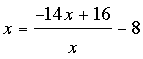SEARCH HOMEMath Central Quandaries & QueriesQuestion from Kory, a student: Solve the equation. x=(-14x+16)/x-8Hi Kory,

To solve this expressionfor x I would first multiply each side by x to eliminate the fraction. That gives me

x2 = - 14x + 16 - 8x

which simplifies to

x2 + 6x - 16 = 0

Can you complete the problem now?

PennyMath Central is supported by the University of Regina and The Pacific Institute for the Mathematical Sciences.